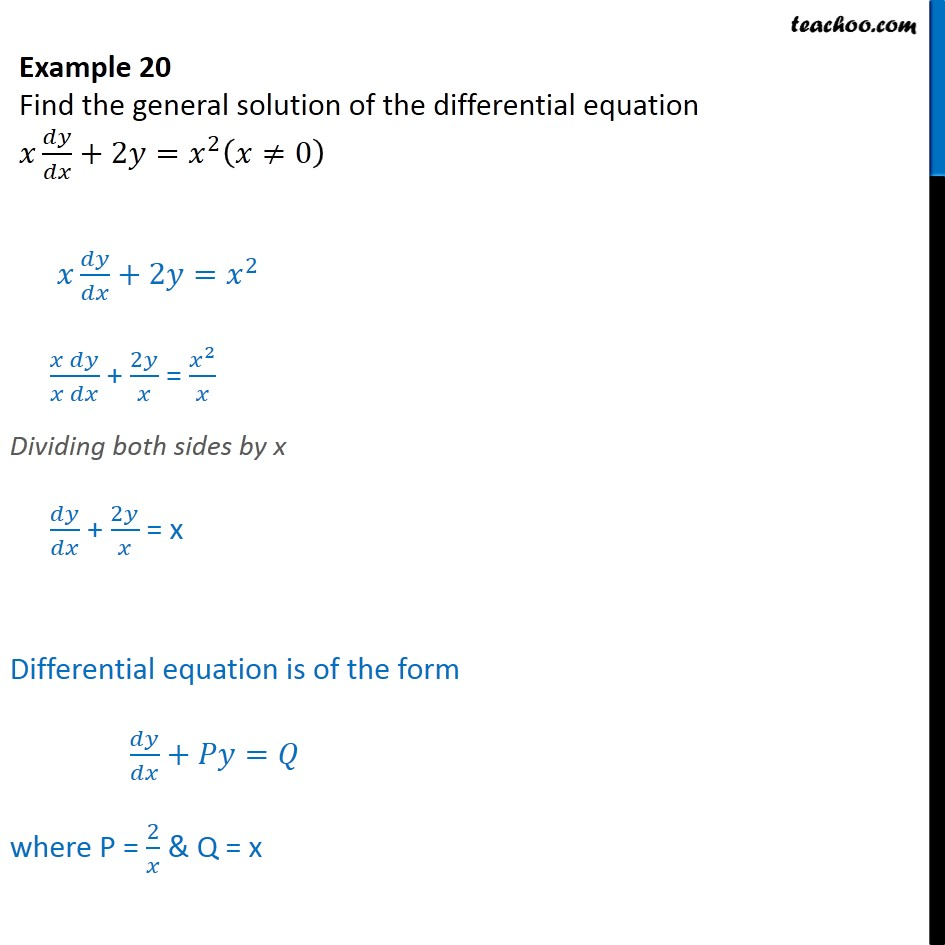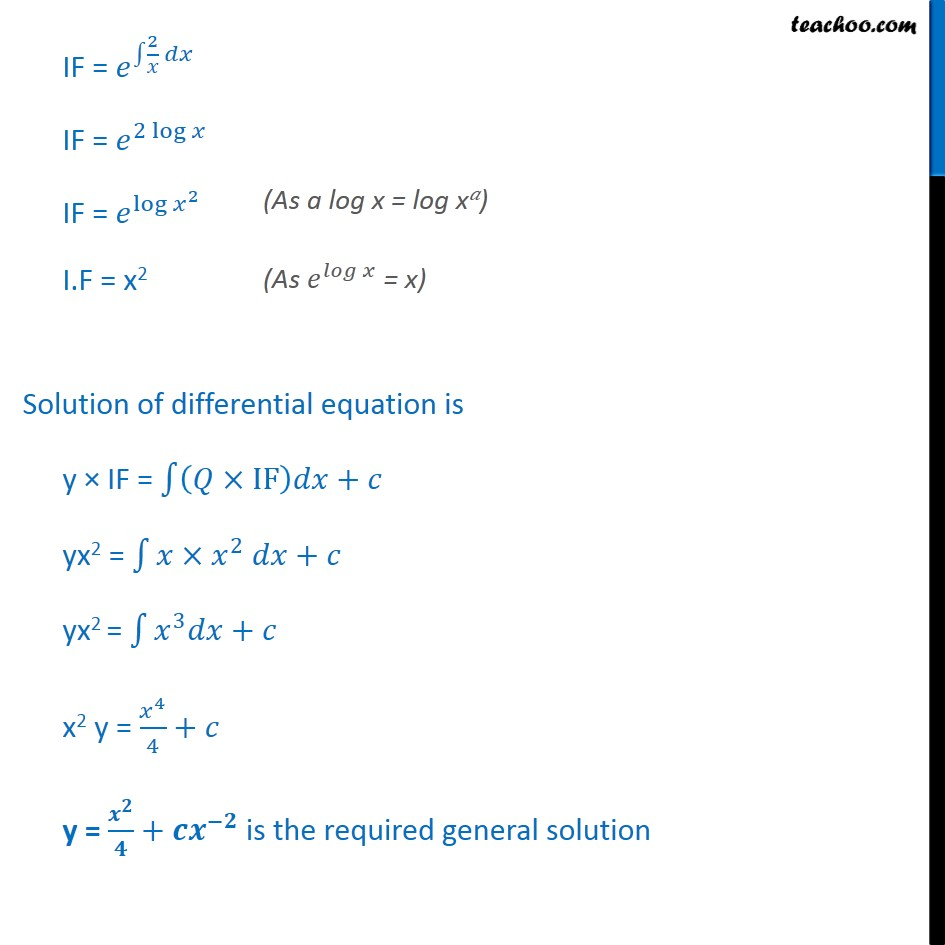Examples

Chapter 9 Class 12 Differential Equations
Serial order wiseLearn in your speed, with individual attention - Teachoo Maths 1-on-1 Class

### Transcript

Example 15 Find the general solution of the differential equation +2 = 2 0 +2 = 2 + 2 = 2 Dividing both sides by x + 2 = x Differential equation is of the form + = where P = 2 & Q = x IF = spdx IF = 2 IF = 2 log IF = log 2 I.F = x2 Solution of differential equation is y IF = IF + yx2 = 2 + yx2 = 3 + x2 y = 4 4 + y = + is the required general solution